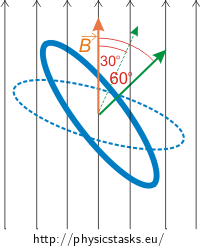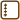## Voltage Induced in a Rotating Circular Loop

### Task number: 1781

A homogeneous magnetic field has a magnetic induction B = 0.5 T. A circular conductive loop with a diameter of 10 cm is placed in the field so that its normal vector forms an angle of 60° with the direction of the B‑field. Determine the average magnitude of the electromotive force induced in the loop during its rotation when the angle changes from 60° to 30° in 0.02 s.• #### Hint

Realize, when electromotive force is induced in a conductive loop and what the connection between the induced electromotive force and the magnetic flux is.

• #### Analysis

Electromotive force is induced in a loop when the magnetic flux through the area of the loop changes. The magnetic flux can be visualized as “the number of magnetic field lines that pass through the area of the loop”.

In our case, an angle of 60° is formed between the loop and the magnetic field. We assess the angle between the magnetic field and the loop as the angle formed between the direction of the magnetic field lines and the line perpendicular to the area of the loop. This means that the number of magnetic field lines that pass through the area of the loop in our case is lower than it would be if the loop formed a zero angle with the magnetic field.

The angle formed between the perpendicular to the loop and the field changes from 60° (full lines in the Figure) to 30° (dashed lines in the Figure). A different (higher) number of magnetic field lines passes through the loop in the new (dashed) orientation. An electromotive force thus must be induced in the loop.

The magnitude of the electromotive force induced in a conductive loop is equal to the change of the magnetic flux in time. We calculate the magnetic fluxes in both orientations of the loop and divide the magnitude of their difference by the time that the rotation took. Thus we obtain the average induced electromotive force.

• #### Solution

It was pointed out already in the analysis that an electromotive force is induced in a conductive loop when the magnetic flux through the area of the loop changes. The magnetic flux through the loop changes due to the changing angle between the loop and the direction of the B‑field in our case.

The relation for the calculation of the induced electromotive force is:

$\left|\mathcal{E}\right|=\left|\frac{\Delta \Phi_{B}}{\Delta t}\right|,$

where for ΔΦB we substitute the difference of the fluxes in the two orientations of the loop.

We use the absolute value of the difference because we are interested in the magnitude of the induced electromotive force only:

$|\Delta \Phi_B|= |\Phi_{B2} - \Phi_{B1}|.$

The fluxes in individual orinetations are given by the following formulae:

$\Phi_{B1} = B S \,\cos \alpha_1= B \pi r^{2}\,\cos \alpha_1,$ $\Phi_{B2} = B S \,\cos \alpha_2= B \pi r^{2}\,\cos \alpha_2.$

We substitute the formulae into the relation for the electromotive force:

$|\mathcal{E}|=\frac{|\Phi_{B2} - \Phi_{B1}|}{\Delta t}=\frac{B\pi r^{2}|\cos \alpha_2 -\cos\alpha_1|}{\Delta t}.$
• #### Notation and numerical substitution

 $$B=\,0.5\,\mathrm{T}$$ magnetic B‑field $$r=\,0.05\,\mathrm{m}$$ radius of the circular loop $$\alpha_1=\,60^{\mathrm{\circ}}$$ the initial angle between the loop and the B‑field $$\alpha_2=\,30^{\mathrm{\circ}}$$ the final angle between the loop and the B‑field $$\Delta t=\,0.02\,\mathrm{s}$$ the time taken to change the angle between the loop and the B‑field $$\mathcal{E}=\mathrm{?}\,\mathrm{(V)}$$ the magnitude of the average induced electromotive force during the rotation of the loop

$\mathcal{E}=\frac{B\pi r^{2}\left|\cos 60^{\circ} -\cos30^{\circ}\right|}{\Delta t}=\frac{0.5\,\cdot\,\pi \,\cdot\,0.05^{2}\,\cdot\,\left|\frac{1}{2} -\frac{\sqrt{3}}{2}\right|}{ 0.02}\,\mathrm{V}=0.07\,\mathrm{V}$
• #### Answer

The average magnitude of the electromotive force induced in the conductive loop is  = 0.07 V.

• #### Comment - Course of the Induced Electromotive Force during Steady Rotation

Our aim is to assess the course of the induced eletromotive force in addition to its average value. For this we need to assess the course of the magnetic flux through the loop in time because the magnitude of the electromotive force induced in the conductive loop is equal to the speed of change (i.e. the time derivative) of the magnetic flux passing through the loop:

$|\mathcal{E}| = \left|\frac{\mathrm{d}\Phi_B}{\mathrm{d}t}\right|.$

1. First, we determine the course of the angle between the loop and the magnetic field. Let us suppose that the loop rotates steadily between positions 1 and 2. The angle α between the normal of the loop and magnetic field lines is decreasing in time t linearly from the initial angle α1:

$\alpha =\alpha_1 - \omega t,$

where ω is the angular frequency of the rotation. The angular frequency must be so that in time $$t=0.02\,\mathrm{s}$$, the angle reaches the final value α2

$\alpha_2=\alpha_1-\omega\cdot0.02$

We substitute the two angles in radians to calculate the angular frequency. Remember that $$60°=\frac{\pi}{3}$$,$$30°=\frac{\pi}{6}$$

$\frac{\pi}{6}= \frac{\pi}{3}-\omega \cdot 0.02.$

And we express the angular frequency:

$\omega = \frac{\pi}{6 {\cdot} 0.02} = \frac{\pi}{0.12} \, \mathrm{s^{-1}} \\ \left( \omega \doteq 26.18\,\mathrm{rad \cdot s^{-1}}, \,\, f = \frac{\omega}{2\pi} \doteq 4.17 \, \mathrm{Hz} \right) .$

2. Second, we calculate the time derivative of the magnetic flux. We substitute our dependence of angle on time into the definition of the magnetic flux

$\Phi_B = B \cdot S \cdot \cos \alpha = B \cdot S \cdot \cos(\alpha_1 -\omega t).$

and differentiate it according to the above mentioned definition of the electromotive force:

$|\mathcal{E}|= \left|\frac{\mathrm{d}\Phi_B}{\mathrm{d}t}\right|.$

(We are intereseted in the magnitude of the induced electromotive force. For this reason we use the absolute values in the formula.) We substitute the expression for the magnetic flux into the formula:

$|\mathcal{E}|=\left|\frac{\mathrm{d}}{\mathrm{d}t}(B \cdot S \cdot \cos\,(\alpha_1-\omega t))\right|,$

Only the expression $$cos(\alpha_1 - \omega t)$$ is a function of time, the other quantitites are constants that do not depend on time and we can factor them out in front of the derivative:

$|\mathcal{E}|=\left| B \cdot S \cdot \frac{\mathrm{d}}{\mathrm{d}t}(\cos\,(\alpha_1-\omega t))\right|$

The derivative of the composed function is equal to the product of the derivative of the inner function (α1-ωt), which is (− ω), and of the derivative of the outer cosine, which is (− sine):

$|\mathcal{E}|=\left| B \cdot S \cdot (-\omega) \cdot (-\sin\,(\alpha_1-\omega t))\right|.$

We arrange the expression and remove the absolute values from the positive quantities:

$|\mathcal{E}| = B \cdot S \cdot \omega \cdot |\sin(\alpha_1-\omega t)|.$

When we substitute the numerical values, we obtain the general function describing the magnitude of the induced electromotive force in time

\begin{align} |\mathcal{E}| &= 0.5 \cdot \pi \cdot 0.05^2 \frac{\pi}{0.12} × \left| \sin \left( \frac{\pi}{3} - \frac{\pi}{0.12} t \right) \right| = \\ &= \frac{125 \cdot \pi^2}{12} \cdot 10^{-3} × \left| \sin \left( \frac{\pi}{3} - \frac{\pi}{0.12} t \right) \right|. \end{align}

The electromotive force induced in the loop at t = 0 s is

$\left| \mathcal{E}_{t \, = \, 0 \mathrm{s}} \right| = \frac{125 \cdot \pi^2}{12} \cdot 10^{-3} × \left| \sin \left( \frac{\pi}{3} - \frac{\pi}{0.12} \cdot 0 \right) \right| \doteq 0.089 \, \mathrm{V}$

and at t = 0.02 s it is

$\left| \mathcal{E}_{t \, = \, 0.02 \mathrm{s}} \right| = \frac{125 \cdot \pi^2}{12} \cdot 10^{-3} × \left| \sin \left( \frac{\pi}{3} - \frac{\pi}{0.12} \cdot 0.02 \right) \right| \doteq 0.051 \, \mathrm{V}.$

The magnitude of the electromotive force that is induced in the rotating conductive loop decreases from the value of 0.089 V at 60° to 0.051 V at 30°.